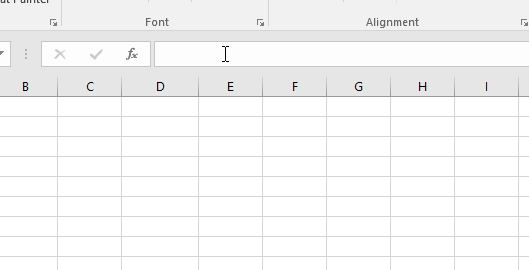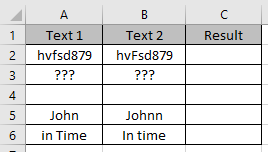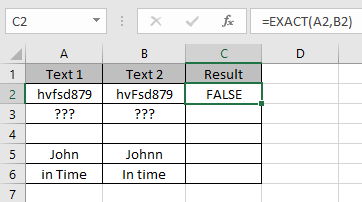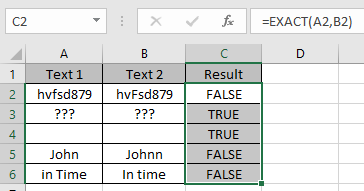# How to use EXACT function in Microsoft Excel

In simple words, this function only returns True when the values are an exact match with each other.

EXACT function returns True or False based on the Exact match or not.
Syntax:

=EXACT (“text1”, “text2”)

Text1 : first value
Text2 : second value

Let’s understand this function using it in an example.The formula returns the True as the two texts are exact same.

Here we have a list of text 1 & text 2.Use the formula:
=EXACT(A2,B2)
A2 : first value
B2 : second valueAs you can see the texts aren’t exact. So it returns False.

Copy the formula in the cell using shortcut key Ctrl + DAs you can see the EXACT function returns True only if both values are an exact match.

Hope you understood how to use Indirect function and referring cell in Excel. Explore more articles on Excel function here. Please feel free to state your query or feedback for the above article.

Popular Articles:

How to use the VLOOKUP Function in Excel

How to use the COUNTIF function in Excel 2016

How to Use SUMIF Function in Excel

Terms and Conditions of use

The applications/code on this site are distributed as is and without warranties or liability. In no event shall the owner of the copyrights, or the authors of the applications/code be liable for any loss of profit, any problems or any damage resulting from the use or evaluation of the applications/code.